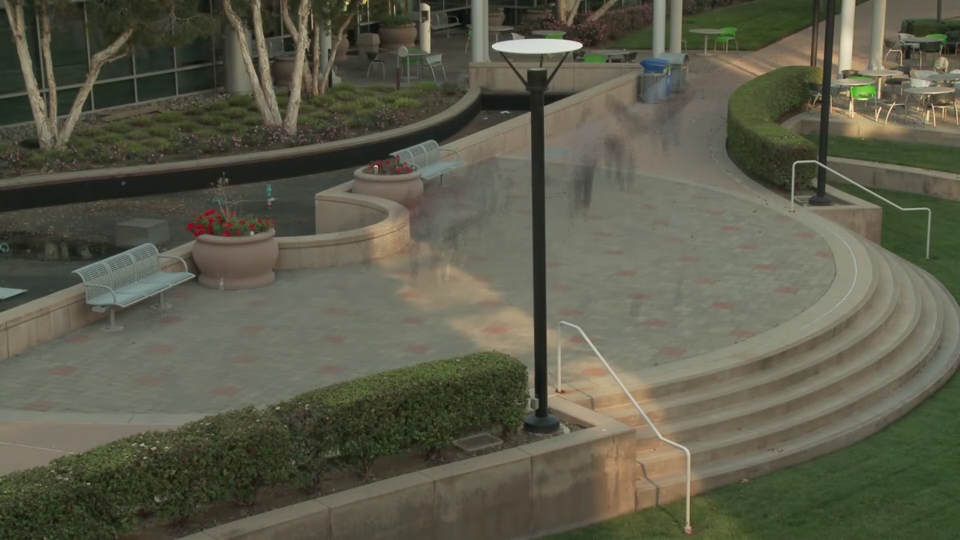## VPI - Vision Programming Interface

#### 2.3 Release

Background Subtractor

# Overview

Background subtraction is an algorithm which is used to separate the foreground objects from the background image in the continuous video sequence. By feeding the frames from the video as input, the binary (or ternary, if including shadow regions) foreground mask and an estimate of the static background image (if enabled) will be produced.# Implementation

For each pixel, a group of gaussian models will be created based on the illumination variations of the video sequence. For a pixel from the image, the GMM can be described as:

$p(\vec{x}) = \sum_{m=1}^{M}\pi_mN(\vec{x};\vec{\mu}_m, {\vec{\delta}_m}^2)$

where:
$$\vec{x}$$ is the pixel value
$$M$$ is the total number of gaussian models
$$\pi_m$$ is the weight corresponding to m-th gaussian model
$$\vec{\mu}_m$$ is the mean of m-th gaussian model
$$\vec{\delta}_m$$ is the standard variance of m-th gaussian model

Mahalanobis distance is used to measure of the distance between a point and a distribution. For more information, see . Here we denote $$D(\vec{x}, m)$$ as the Mahalanobis distance between the pixel value x and m-th model.

For any new pixel from the video sequence, it will calculate the Mahalanobis distance $$D(\vec{x}, m)$$ against each gaussian model. If the distance is within the threshold, then the model is found. Otherwise it will create new gaussian model if the maximum number of models has not been reached. Based on the weight of the matched model, it will classify the pixel as foreground or background. For more information, see .

# C API functions

For list of limitations, constraints and backends that implements the algorithm, consult reference documentation of the following functions:

Function Description
vpiInitBackgroundSubtractorParams Initializes VPIBackgroundSubtractorParams with default values.
vpiSubmitBackgroundSubtractor Submits a Background Subtractor operation to the stream.

# Usage

Language:
1. Import VPI module
import vpi
2. Initialization phase
1. Create the Background Subtractor object, configuring it to handle RGB8 inputs with dimensions size. The CUDA backend will be used to execute the algorithm.
with vpi.Backend.CUDA:
bgsub = vpi.BackgroundSubtractor(size, vpi.Format.RGB8)
3. Processing phase
1. Fetch a new frame from input video sequence. Here it's assumed that it's already VPI image with RGB8 format.
2. Feed it into the bgsub object. The estimation of the foreground mask and the background are returned as VPI images.
1. Initialization phase:
1. Include the header that defines the background subtractor function:
Declares functions that implement background subtractor algorithms.
2. Define the required images:
VPIImage input = /* frame from the video sequence */;
VPIPyramid fgmask = /* foreground mask. Format has to be VPI_IMAGE_FORMAT_U8 */;
VPIPyramid bgimage = /* background image. Image format has to be the same as input image format */;
struct VPIImageImpl * VPIImage
A handle to an image.
Definition: Types.h:256
struct VPIPyramidImpl * VPIPyramid
A handle to an image pyramid.
Definition: Types.h:262
3. Create the stream to which the algorithm is to be submitted for execution:
VPIStream stream;
vpiStreamCreate(0, &stream);
struct VPIStreamImpl * VPIStream
A handle to a stream.
Definition: Types.h:250
VPIStatus vpiStreamCreate(uint64_t flags, VPIStream *stream)
Create a stream instance.
4. Create the algorithm payload to process input image with provided width, height and format:
#define VPI_IMAGE_FORMAT_RGB8
Single plane with interleaved RGB 8-bit channel.
Definition: ImageFormat.h:287
A handle to an algorithm payload.
Definition: Types.h:268
@ VPI_BACKEND_CUDA
CUDA backend.
Definition: Types.h:93
2. Processing phase:
1. Start of the processing loop. Fetch the input from the video sequence.
for (i = 0; i < nframes; ++i)
{
input = /* Fetch frame from video sequence */;
2. Configure the parameters for the submission:
algoParams.learningRate = 0.01;
float learningRate
Learning rate that indicates how fast the background model is learnt.
VPIStatus vpiInitBackgroundSubtractorParams(VPIBackgroundSubtractorParams *params)
Initializes VPIBackgroundSubtractorParams with default values.
Structure that defines the parameters for vpiCreateBackgroundSubtractor.
3. Submit the algorithm to the stream, to be executed by the CUDA backend, along with current input frame, the output foreground mask and background image:
Submits a Background Subtractor operation to the stream.
4. Optionally, wait until the processing is done:
vpiStreamSync(stream);
VPIStatus vpiStreamSync(VPIStream stream)
Blocks the calling thread until all submitted commands in this stream queue are done (queue is empty)...
5. Process the results once they are ready:
}
3. Cleanup phase:
1. Free resources held by the stream, the payload, the input image, the foreground image and background image:
vpiImageDestroy(bgimage);
void vpiImageDestroy(VPIImage img)
Destroy an image instance.
Deallocates the payload object and all associated resources.
void vpiStreamDestroy(VPIStream stream)
Destroy a stream instance and deallocate all HW resources.

# Performance

For information on how to use the performance table below, see Algorithm Performance Tables.
Before comparing measurements, consult Comparing Algorithm Elapsed Times.
For further information on how performance was benchmarked, see Performance Benchmark.

-

## References

1. Z. Zivkovic, "Improved Adaptive Gaussian Mixture Model for Background Subtraction."
Proceedings of the 17th International Conference on Pattern Recognition, 2004. ICPR 2004.
2. Wikipedia article on Mahalanobis distance